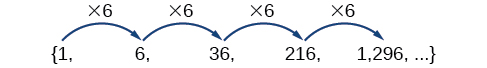# 11.3 Geometric sequences

 Page 1 / 6
In this section, you will:
• Find the common ratio for a geometric sequence.
• List the terms of a geometric sequence.
• Use a recursive formula for a geometric sequence.
• Use an explicit formula for a geometric sequence.

Many jobs offer an annual cost-of-living increase to keep salaries consistent with inflation. Suppose, for example, a recent college graduate finds a position as a sales manager earning an annual salary of $26,000. He is promised a 2% cost of living increase each year. His annual salary in any given year can be found by multiplying his salary from the previous year by 102%. His salary will be$26,520 after one year; $27,050.40 after two years;$27,591.41 after three years; and so on. When a salary increases by a constant rate each year, the salary grows by a constant factor. In this section, we will review sequences that grow in this way.

## Finding common ratios

The yearly salary values described form a geometric sequence because they change by a constant factor each year. Each term of a geometric sequence increases or decreases by a constant factor called the common ratio . The sequence below is an example of a geometric sequence because each term increases by a constant factor of 6. Multiplying any term of the sequence by the common ratio 6 generates the subsequent term.## Definition of a geometric sequence

A geometric sequence    is one in which any term divided by the previous term is a constant. This constant is called the common ratio    of the sequence. The common ratio can be found by dividing any term in the sequence by the previous term. If ${a}_{1}$ is the initial term of a geometric sequence and $r$ is the common ratio, the sequence will be

Given a set of numbers, determine if they represent a geometric sequence.

1. Divide each term by the previous term.
2. Compare the quotients. If they are the same, a common ratio exists and the sequence is geometric.

## Finding common ratios

Is the sequence geometric? If so, find the common ratio.

1. $1\text{,}\text{\hspace{0.17em}}2\text{,}\text{\hspace{0.17em}}4\text{,}\text{\hspace{0.17em}}8\text{,}\text{\hspace{0.17em}}16\text{,}\text{\hspace{0.17em}}...$

Divide each term by the previous term to determine whether a common ratio exists.

1. $\begin{array}{llllllllll}\frac{2}{1}=2\hfill & \hfill & \hfill & \frac{4}{2}=2\hfill & \hfill & \hfill & \frac{8}{4}=2\hfill & \hfill & \hfill & \frac{16}{8}=2\hfill \end{array}$

The sequence is geometric because there is a common ratio. The common ratio is 2.

2. $\begin{array}{lllllll}\frac{12}{48}=\frac{1}{4}\hfill & \hfill & \hfill & \frac{4}{12}=\frac{1}{3}\hfill & \hfill & \hfill & \frac{2}{4}=\frac{1}{2}\hfill \end{array}$

The sequence is not geometric because there is not a common ratio.

If you are told that a sequence is geometric, do you have to divide every term by the previous term to find the common ratio?

No. If you know that the sequence is geometric, you can choose any one term in the sequence and divide it by the previous term to find the common ratio.

Is the sequence geometric? If so, find the common ratio.

$5,10,15,20,...$

The sequence is not geometric because $\frac{10}{5}\ne \frac{15}{10}$ .

Is the sequence geometric? If so, find the common ratio.

$100,20,4,\frac{4}{5},...$

The sequence is geometric. The common ratio is $\frac{1}{5}$ .

## Writing terms of geometric sequences

Now that we can identify a geometric sequence, we will learn how to find the terms of a geometric sequence if we are given the first term and the common ratio. The terms of a geometric sequence can be found by beginning with the first term and multiplying by the common ratio repeatedly. For instance, if the first term of a geometric sequence is ${a}_{1}=-2$ and the common ratio is $r=4,$ we can find subsequent terms by multiplying $-2\cdot 4$ to get $-8$ then multiplying the result $-8\cdot 4$ to get $-32$ and so on.

what is set?
a colony of bacteria is growing exponentially doubling in size every 100 minutes. how much minutes will it take for the colony of bacteria to triple in size
I got 300 minutes. is it right?
Patience
no. should be about 150 minutes.
Jason
It should be 158.5 minutes.
Mr
ok, thanks
Patience
100•3=300 300=50•2^x 6=2^x x=log_2(6) =2.5849625 so, 300=50•2^2.5849625 and, so, the # of bacteria will double every (100•2.5849625) = 258.49625 minutes
Thomas
what is the importance knowing the graph of circular functions?
can get some help basic precalculus
What do you need help with?
Andrew
how to convert general to standard form with not perfect trinomial
can get some help inverse function
ismail
Rectangle coordinate
how to find for x
it depends on the equation
Robert
yeah, it does. why do we attempt to gain all of them one side or the other?
Melissa
whats a domain
The domain of a function is the set of all input on which the function is defined. For example all real numbers are the Domain of any Polynomial function.
Spiro
Spiro; thanks for putting it out there like that, 😁
Melissa
foci (–7,–17) and (–7,17), the absolute value of the differenceof the distances of any point from the foci is 24.
difference between calculus and pre calculus?
give me an example of a problem so that I can practice answering
x³+y³+z³=42
Robert
dont forget the cube in each variable ;)
Robert
of she solves that, well ... then she has a lot of computational force under her command ....
Walter
what is a function?
I want to learn about the law of exponent
explain this
what is functions?
A mathematical relation such that every input has only one out.
Spiro
yes..it is a relationo of orders pairs of sets one or more input that leads to a exactly one output.
Mubita
Is a rule that assigns to each element X in a set A exactly one element, called F(x), in a set B.
RichieRich

#### Get Jobilize Job Search Mobile App in your pocket Now!ByByByBy Brenna FikeBy DanielrosenbergerBy OpenStaxBy OpenStaxBy OpenStaxBy OpenStaxBy Richley CrapoBy Anh DaoBy Abby Sharp